Refer to our Texas Go Math Grade 2 Answer Key Pdf to score good marks in the exams. Test yourself by practicing the problems from Texas Go Math Grade 2 Lesson 10.3 Answer Key 3-Digit Addition: Regroup Tens.

Explore

Use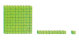to model the problem. Draw quick pictures to show what you did.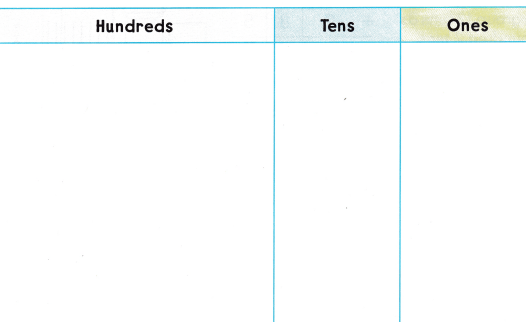FOR THE TEACHER • Read the following problem and have children model it with blocks. On Monday, 253 children visited the aquarium. On Tuesday, 324 children visited the aquarium. How many children visited the aquarium those two days? Have children draw quick pictures to show how they solved the problem.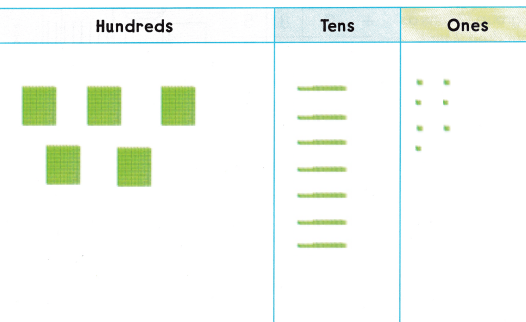Math Talk
Mathematical Processes

Explain how your quick pictures show what happened in the problem.
The sum of 253 and 324 is 567
So, i drew 5 hundred blocks, 6 ten blocks and 7 one blocks.
Model and Draw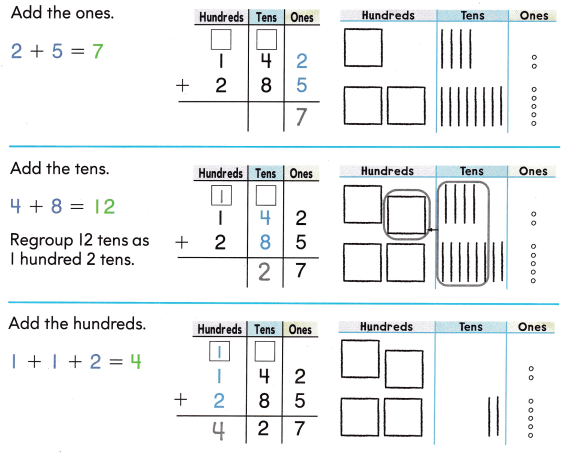Share and Show

Write the sum.

Question 1.Explanation:
The sum of 347 and 291 is 638.

Question 2.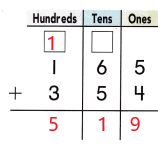Explanation:
The sum of 165 and 354 is 519.

Question 3.Explanation:
The sum of 538 and 140 is 678.

Problem Solving

Write the sum.

Question 4.Explanation:
The sum of 224 and 157 is 381.

Question 5.Explanation:
The sum of 254 and 405 is 659.

Question 6.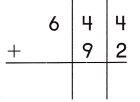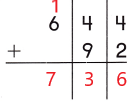Explanation:
The sum of 644 and 92 is 736.

Question 7.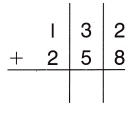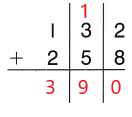Explanation:
The sum of 132 and 258 is 390.

Question 8.Explanation:
The sum of 314 and 435 is 749.

Question 9.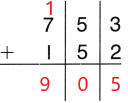Explanation:
The sum of 753 and 152 is 905.

Solve. Write or draw to explain.

Question 10.
H.O.T. These lists show the pieces of fruit sold. How many pieces of fruit did Mr. Olson sell?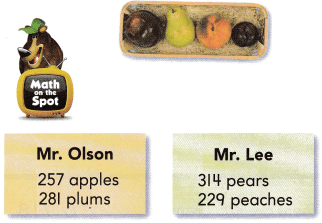___________ pieces of fruitExplanation:
The sum of 257 and 281 is 538
Mr. Olson has 538 pieces of fruit.

Question 11.
H.O.T. Multi-Step Who sold more pieces of fruit? How many more?
____________ more pieces of fruit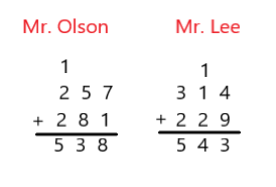Explanation:
Mr. Lee sold 5 more pieces of fruits than Mr. Olson.

Question 12.
The state of Washington has 142 state parks. The state of Michigan has 98 state parks. How many state parks do these two states have?
(A) 238
(B) 140
(C) 240
(C) 240

Explanation:
The state of Washington has 142 state parks
The state of Michigan has 98 state parks
142 + 98 = 240
So, These states have 240 state parks.

Question 13.
Analyze In the morning, 972 people visit the park. In the afternoon, 939 people visit. How many people in all visit the park during the day?
(A) 1919
(B) 1911
(C) 1901
(B) 1911

Explanation:
In the morning, 972 people visit the park
In the afternoon 939 people visit
972 + 939 = 1911
So, 1911 people visited the park during the day in all.

Question 14.
Record On Saturday, 365 children visit a museum. On Sunday, 277 children visit the museum. How many children visit the museum on the two days?
(A) 642
(B) 547
(C) 637
(A) 642

Explanation:
On Saturday, 365 children visit a museum
On Sunday 277 children visit the museum
365 + 277 = 642
So, 642 children visited the museum on the two days.

Question 15.
Multi-Step In a bowling game, Jack scored 116 points and 124 points. Hal scored 128 points and 134 points. Who scored more points? How many more points were scored?(A) 718
(B) 698
(C) 708

TAKE HOME ACTIVITY • Hove your child explain to you how to find the sum for 45 + 73.

### Texas Go Math Grade 2 Lesson 10.3 Homework and Practice Answer Key

Write the sum.

Question 1.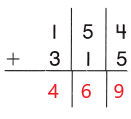Explanation:
The sum of 154 and 315 is 469.

Question 2.Explanation:
The sum of 664 and 73 is 737.

Question 3.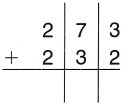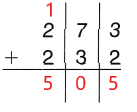Explanation:
The sum of 273 and 232 is 505.

Question 4.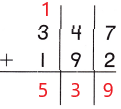Explanation:
The sum of 347 and 192 is 539.

Question 5.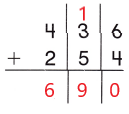Explanation:
The sum of 436 and 254 is 690.

Question 6.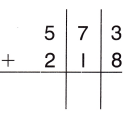Explanation:
The sum of 573 and 218 is 791.

Problem Solving

Solve.

Question 7.
MultiStep: In a bowling game, Jack scored 116 points and l2 points. Hal scored 128 points and 134 points. Who scored more points? How many more points were scored?

Lesson Check

Question 8.
There are 288 potatoes packed in crates. There are 160 potatoes in plastic bags. How many potatoes are there?
(A) 448
(B) 428
(C) 348
(A) 448

Explanation:
There are 288 potatoes packed in crates
There are 160 potatoes in plastic bags
288 + 160 = 448
So, there are 448 potatoes.

Question 9.
Bennett Farm has 334 apple trees. Nolan Farm has 382 apple trees. How many apple trees do these two farms have?
(A) 616
(B) 716
(C) 706
(B) 716

Explanation:
Bennett Farm has 334 apple trees
Nolan Farm has 382 apple trees
334 + 382 = 716
So, there are 716 apple trees in two farms.

Question 10.
In the morning, 374 people visit the aquarium. In the afternoon, 530 people visit. How many people visit the aquarium during the day?
(A) 804
(B) 914
(C) 904
(C) 904

Explanation:
In the morning, 374 people visit the aquarium
In the afternoon, 530 people visit
374 + 530 = 904
So, 904 people visited the aquarium during the day.

Question 11.
There are two schools in the town of Madison. Memorial School has 453 students. Lincoln School has 438 students. How many students go to school in town?
(A) 885
(B) 891
(C) 881